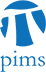## SCAIM Seminar: How fast can we multiply and divide polynomials?

• Date: 10/27/2009
Lecturer(s):
Michael Monagan (Simon Fraser University)
Location:

University of British Columbia

Description:

Computer algebra systems like Maple and Mathematica spend most of their time doing either polynomial arithmetic or linear algebra. For polynomials in one variable of degree n, the Fast Fourier Transform gives us an O(n log n) multiplication algorithm. But for polynomials in several variables, which are usually sparse (most of the coefficients are zero) there are no O(n log n) algorithms. So what's the best way to multiply and divide them?

In the talk I will present

* Johnson's algorithm from 1974 for multiplying two sparse polynomials using a heap.

* Our division algorithm which also uses a heap.

* Some benchmarks showing that these algorithms are 100 times faster than Maple and Mathematica, and

* a parallel algorithm for multiplication using a heap.

I'd also like to present an optimization which we call "immediate monomials" where we reduce multiplication of monomials ( x^i y^j z^k ) to one machine instruction and describe a new project we are doing with Maple to redesign the basic polynomial representation in Maple to hopefully get a good overall speedup.

This is joint work with Roman Pearce

Schedule:

12:30 - 14:00, WMAX 216.

Pizza and pop will be provided!

Other Information:

For details, please visit the official website at